This resource function is obsolete. Use the paclet Wolfram/QuantumFramework instead.

Function Repository Resource:

# QuantumDistance

Compute the Hilbert space distance between two discrete quantum states

Contributed by: Jonathan Gorard
 ResourceFunction["QuantumDistance"][QuantumDiscreteState[qds1,…],QuantumDiscreteState[qds2,…]] returns the fidelity distance (defined below) between the discrete quantum states qds1 and qds2. ResourceFunction["QuantumDistance"][QuantumDiscreteState[qds1,…],QuantumDiscreteState[qds2,…],measure] returns the distance using the Hilbert space distance measure measure.

## Details

ResourceFunction["QuantumDistance"][] returns a (potentially complex) scalar value representing the distance between the specified discrete quantum states.
In ResourceFunction["QuantumDistance"][QuantumDiscreteState[qds1,],QuantumDiscreteState[qds2,]], the "Fidelity" measure is used by default.
In ResourceFunction["QuantumDistance"][QuantumDiscreteState[qds1,],QuantumDiscreteState[qds2,],measure], the following Hilbert space distance measures measure are supported:
 "Fidelity" 1 minus the fidelity of the two states (i.e. 1 minus the most general probability that the two states will measure to be equivalent) "RelativeEntropy" relative von Neumann entropy distance between the two states (i.e. the quantum analog of the Kullback–Leibler divergence) "Trace" trace distance between the density matrices of the two states (i.e. the quantum analog of the Kolmogorov–Smirnov distance) "BuresAngle" infinitesimal Bures angle distance between the density matrices of the two states (i.e. the quantum analog of the Fisher information metric) "HilbertSchmidt" Hilbert–Schmidt information distance between the two states "Bloch" coordinate distance between two qubit states on the Bloch sphere

## Examples

### Basic Examples (4)

Find the default (fidelity) distance between two trivial pure quantum states:

 In:=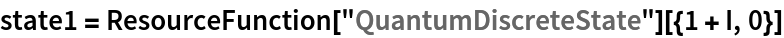Out=In:=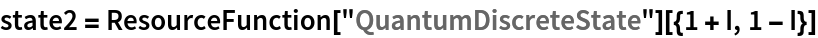Out=In:=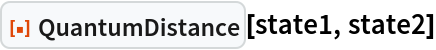Out=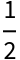Find the fidelity distance between two mixed quantum states:

 In:=Out=Find the default (fidelity) distance between a pure state and a mixed state:

 In:=Out=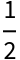Find the trace distance between the same pure state and mixed state:

 In:=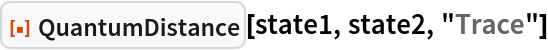Out=Find the coordinate distance between two random pure qubit states on the Bloch sphere:

 In:=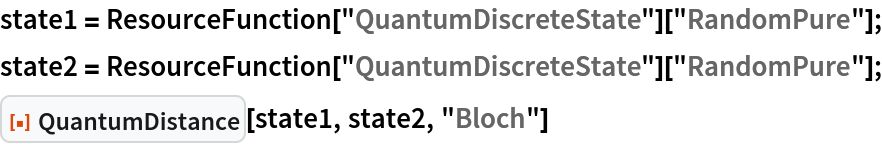Out=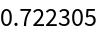Find the relative von Neumann entropy distance between two random 5-dimensional pure states:

 In:=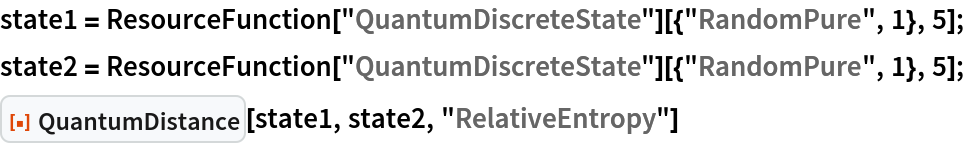Out=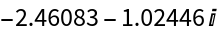### Scope (8)

Find distances between multiqubit states:

 In:=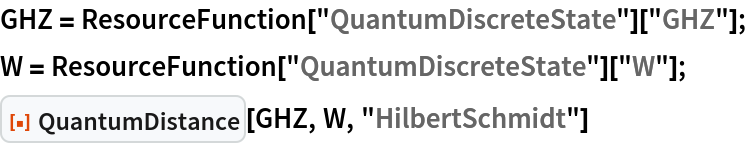Out=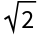Find distances between higher-dimensional qudit states:

 In:=Out=Fidelity distances can be computed between any combination of arbitrary pure and mixed states:

 In:=In:=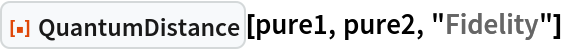Out=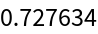In:=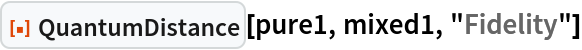Out=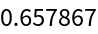In:=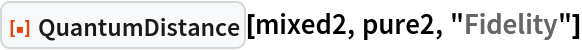Out=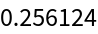In:=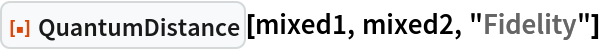Out=Relative entropy distances can be computed between any combination of arbitrary pure and mixed states:

 In:=In:=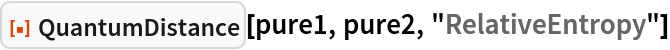Out=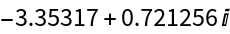In:=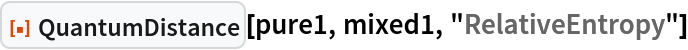Out=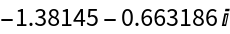In:=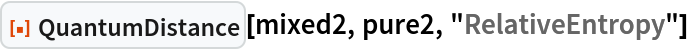Out=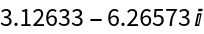In:=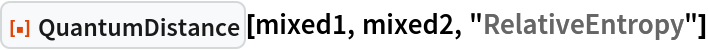Out=Trace distances can be computed between any combination of arbitrary pure and mixed states:

 In:=In:=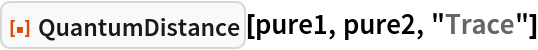Out=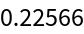In:=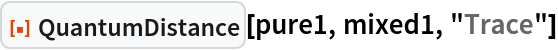Out=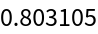In:=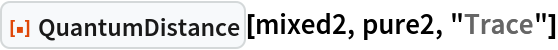Out=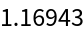In:=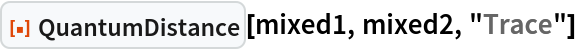Out=Infinitesimal Bures angle distances can be computed between any combination of arbitrary pure and mixed states:

 In:=In:=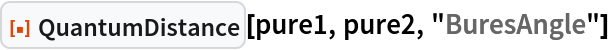Out=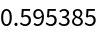In:=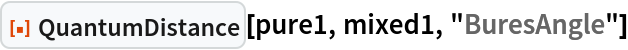Out=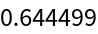In:=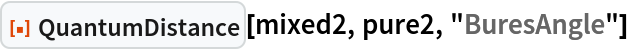Out=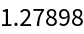In:=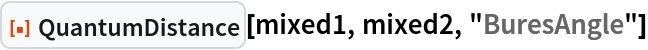Out=Hilbert–Schmidt distances can be computed between any combination of arbitrary pure and mixed states:

 In:=In:=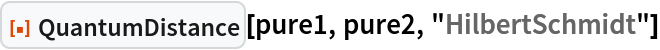Out=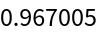In:=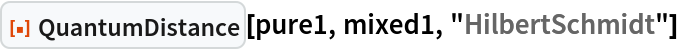Out=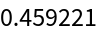In:=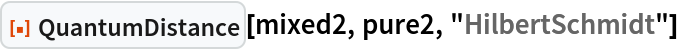Out=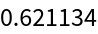In:=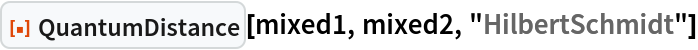Out=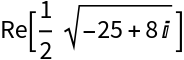Coordinate distances on the Bloch sphere can be computed between any combination of arbitrary pure and mixed qubit (2-dimensional) states:

 In:=In:=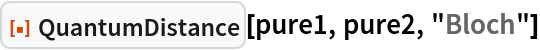Out=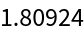In:=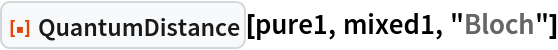Out=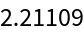In:=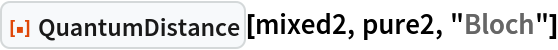Out=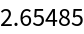In:=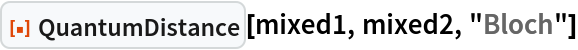Out=However, Bloch distances cannot be computed for arbitrary qudit (higher-dimensional) states (returns unevaluated):

 In:=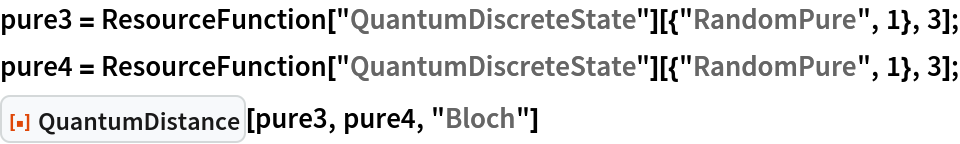Out=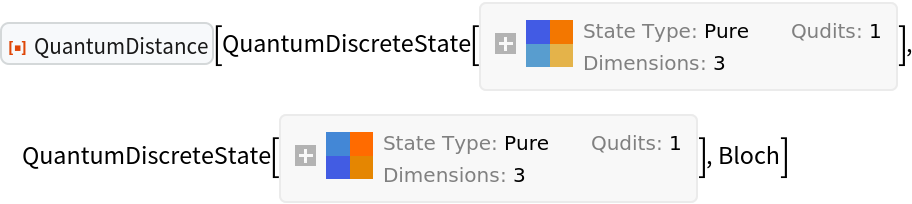Jonathan Gorard

## Version History

• 1.0.0 – 12 April 2021